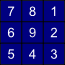# Slope

This tool calculates slope gradient (i.e. slope steepness in degrees) for each grid cell in an input digital elevation model (DEM). The user must specify the name of the input DEM and the output raster image. The Z Conversion Factor is only important when the vertical and horizontal units are not the same in the DEM. When this is the case, the algorithm will multiply each elevation in the DEM by the Z Conversion Factor.

## Algorithm Description

The tool uses Horn's (1981) 3rd-order finite difference method to estimate slope. Given the following clock-type grid cell numbering scheme (Gallant and Wilson, 2000),`slope = arctan(fx2 + fy2)0.5`

where,

`fx = (z3 - z5 + 2(z2 - z6) + z1 - z7)`

and,

`fy = (z7 - z5 + 2(z8 - z4) + z1 - z3)`

## Scripting:

The following is an example of a Python script that uses this tool:

``` wd = pluginHost.getWorkingDirectory() inputFile = wd + "DEM.dep" outputFile = wd + "slope.dep" zFactor = "1.0" args = [inputFile, outputFile, zFactor] pluginHost.runPlugin("Slope", args, False) ```

This is a Groovy script also using this tool:

``` def wd = pluginHost.getWorkingDirectory() def inputFile = wd + "DEM.dep" def outputFile = wd + "slope.dep" def zFactor = "1.0" String[] args = [inputFile, outputFile, zFactor] pluginHost.runPlugin("Slope", args, false) ```

## Credits:

• John Lindsay (2012) email: jlindsay@uoguelph.ca

## References:

• Gallant, J. C., and J. P. Wilson, 2000, Primary topographic attributes, in Terrain Analysis: Principles and Applications, edited by J. P. Wilson and J. C. Gallant pp. 51?86, John Wiley, Hoboken, N.J.